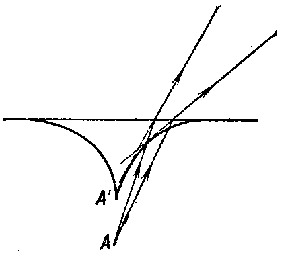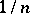# Caustic

(diff) ← Older revision | Latest revision (diff) | Newer revision → (diff)

The envelope of rays reflected or refracted by a given curve. The caustic of reflected rays is called a catacaustic, that of refracted rays — a diacaustic.Figure: c021000aFigure: c021000b

For example, the catacaustic of a parallel beam of rays reflected from a semi-circle is part of an epicycloid (see Fig. a); the diacaustic of a pencil of rays emanating from a pointlying in a denser medium and refracted by a straight line is part of an astroid with cuspthe distance of which from the line istimes that of the point(whereis the refraction index) (see Fig. b).

How to Cite This Entry:
Caustic. Encyclopedia of Mathematics. URL: http://encyclopediaofmath.org/index.php?title=Caustic&oldid=11319
This article was adapted from an original article by A.B. Ivanov (originator), which appeared in Encyclopedia of Mathematics - ISBN 1402006098. See original article